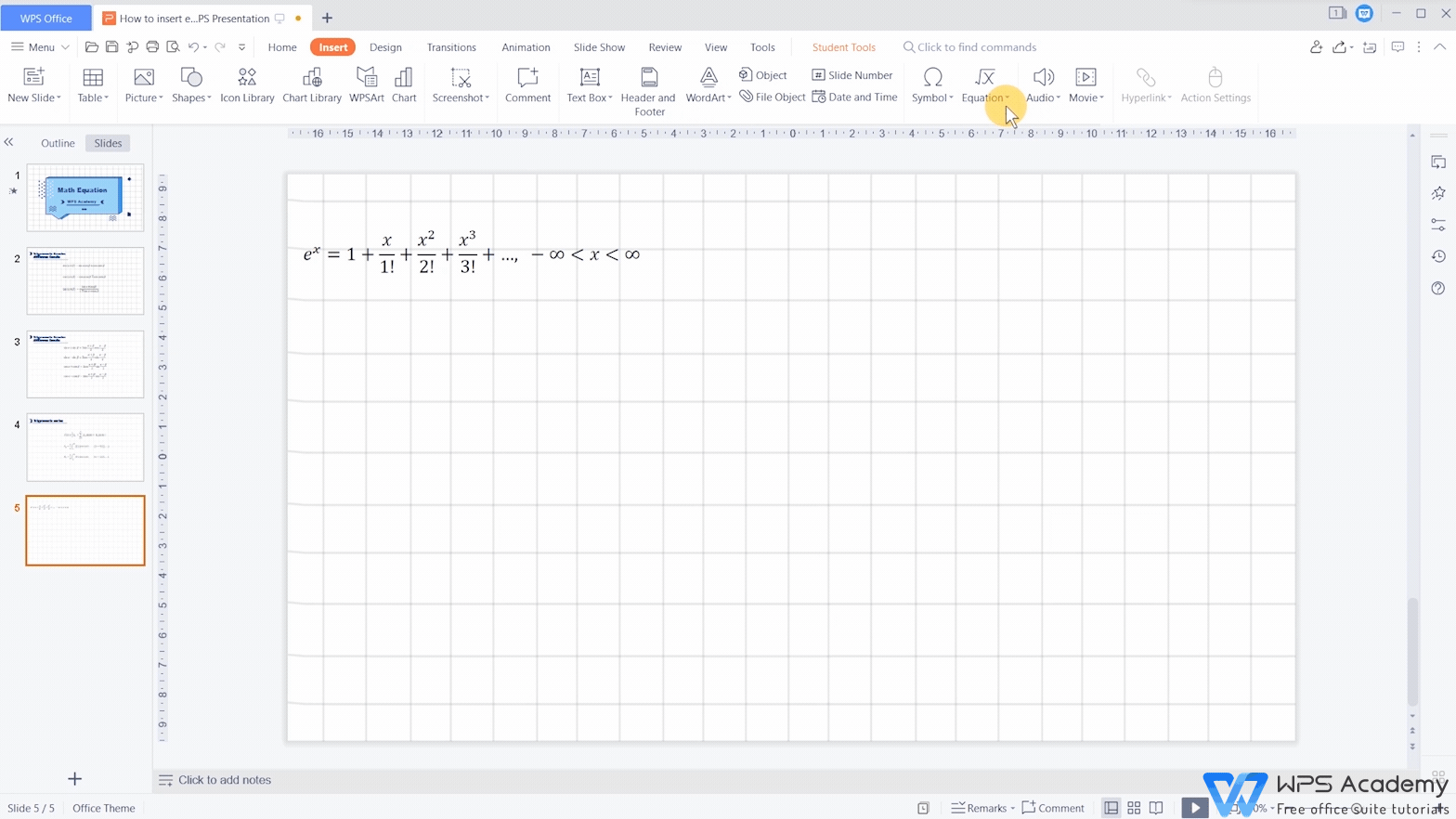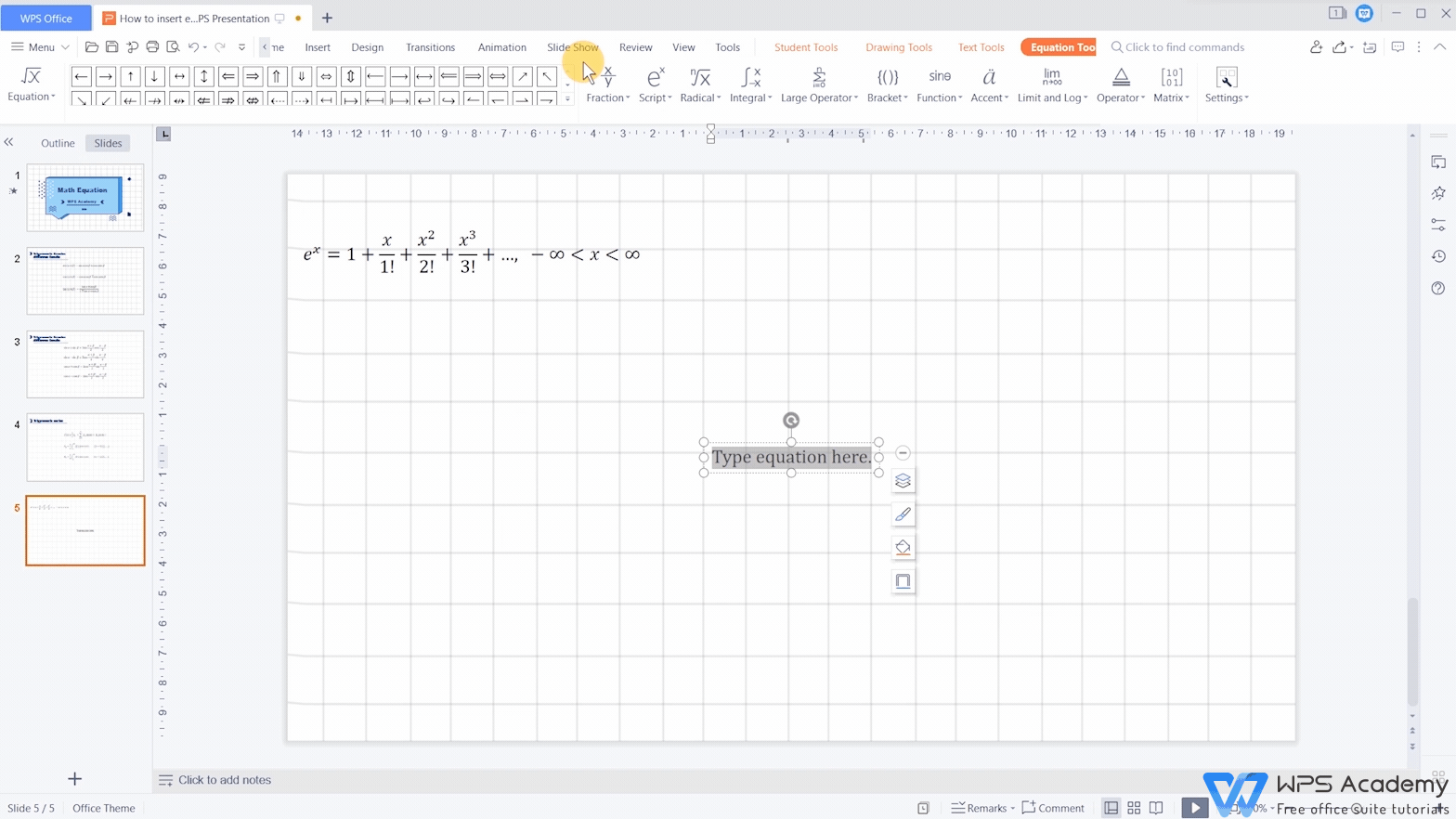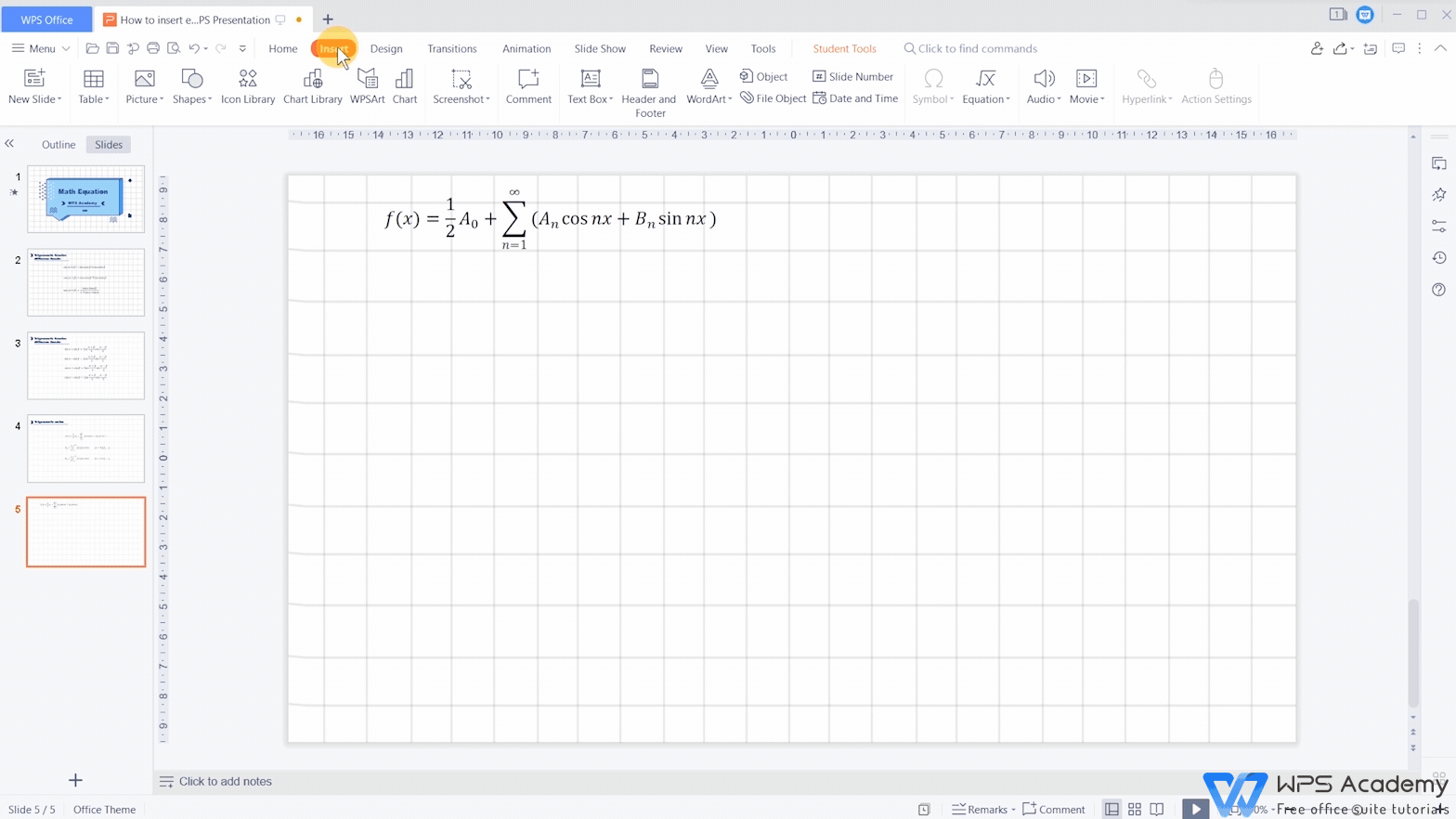# How to insert equations in WPS Presentation

Uploaded time: December 14, 2021 Difficulty: Beginner# How to insert equations in WPS Presentation

You will be the amazing reporter with WPS Presentation, which could be an alternative to Microsoft PowerPoint. Mathematical equations contain a variety of number formats like symbols, scores, root numbers, and so on. It is difficult for us to enter these equations with the input method. However, with the Equation function in WPS Presentation, inserting mathematical equations will no longer be a problem.

Click the Insert tab at the top of the screen, then click the Equation button. Here, WPS Office presets a variety of commonly-used mathematical equations for us, which can be quickly added by clicking.If you can't find what you want in the drop-down menu at Equation, click Insert New Equation” to customize your new equation.

In the Equation Tools tab, we can quickly insert various basic mathematical symbols.Skillfully using Basic Math, we can also choose various symbol classifications, such as Operator, Arrows, and so on.On the right side of the tab menu, we can choose many different number formats, such as Fraction, Subscripts and Superscripts, Radical, Function, etc.

Most mathematical equations can be entered skillfully using the two small functions we mentioned.In addition, we can also use the Equation Editor to edit equations.

Click the Insert tab.Then click the drop-down button of Equation.

Finally, click Equation Editor to open it.

In the pop-up Equation Editor edit box, enter the formula and click Close, The formula will be inserted into the slide as a picture.

Double-click the equation to pop up the Equation Editor again if you want to re-edit.What a practical feature! Did you get it?